# VBA – All Operators Explained

This tutorial will explain the operators used in VBA.

There are a few types of operators in VBA – Arithmetic Operators, Comparison Operators, Logical Operators and Concatenation Operators.

## Arithmetic Operators

These operators allow us to use VBA as a calculator – to add, subtract, multiply, divide and to calculate to the power of (exponentiation).

 Arithmetic Operator Explanation + Addition – Subtraction * Multiplication / Division ^ Exponentiation

The addition or plus sign (+) operator is used to add numbers together in VBA.

``````Sub TestAdd ()
Dim Result As double
Result = 10 + 20
Msgbox "The answer is " & Result
End Sub``````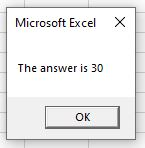### Subtraction

The subtraction or minus sign () operator is used to subtract numbers from each other in VBA.

``````Sub TestMinus ()
Dim Result As Double
Dim A As Integer
Dim B As  Integer
A = 20
B = 10
Result = A - B
Msgbox "The answer is " & Result
End Sub``````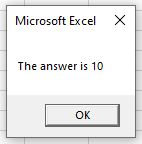### Multiplication

The Multiplication (*) operator is used to multiply numbers together in VBA.

``````Sub TestMultipy ()
Dim Result As Double
Dim A As Integer
Dim B As Integer
A = 20
B = 10
Result = A * B
Msgbox "The answer is " & Result
End Sub``````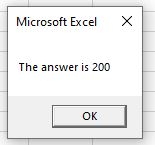### Division

The Division (/) operator is used to divide numbers in VBA

``````Sub TestDivision ()
Dim Result As Double
Dim A As Integer
Dim B As Integer
A = 20
B = 4
Result = A/ B
Msgbox "The answer is " & Result
End Sub``````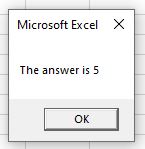Stop searching for VBA code online. Learn more about AutoMacro - A VBA Code Builder that allows beginners to code procedures from scratch with minimal coding knowledge and with many time-saving features for all users!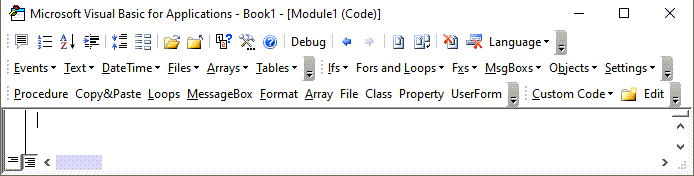### Exponentiation (to the Power of)

The exponentiation  (^) operator is used to calculate a value to the power of a number.

``````Sub TestDivision ()
Dim Result As Double
Dim A As Integer
Dim B As Integer
A = 2
B = 4
Result = A ^ B
Msgbox "The answer is " & Result
End Sub``````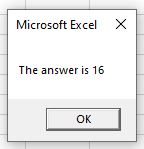## Combining Operators

If we end up having a calculation that combines these operators, remember that the BODMAS (or PEDMAS) order of operators will apply.  In other words the calculation will be done in the order of brackets (parenthesis), power of (exponentiation) , division, multiplication, addition and then subtraction.

``````Sub TestOrder ()
Dim Result As Double
Dim A As Integer
Dim B As Integer
Dim C As Integer
Dim D As Integer
Dim E As Integer
A = 2
B = 10
C = 4
D = 6
E = 2
Result = A+B/C*D^E
Msgbox "The answer is " & Result
End Sub``````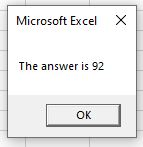The answer above is 92 – first the 6^2 is calculated (36), then the 10 is divided by 4 (2.5).  Next the 2.5 is multiplied by the 36 and finally the 2 is added last.

If we were to add brackets to this calculation, the answer would differ:

``````Sub TestOrder ()
Dim Result As Double
Dim A As Integer
Dim B As Integer
Dim C As Integer
Dim D As Integer
Dim E As Integer
A = 2
B = 10
C = 4
D = 6
E = 2
Result = (A+B/C*D)^E
Msgbox "The answer is " & Result
End Sub
``````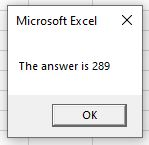## Comparison Operators

Comparison Operators allow you to compare values and return a Boolean True or False as a result.

 Comparison Operator Explanation = Equal to <> Not Equal to > Greater than >= Greater than or Equal to < Less than <= Less than or Equal to

VBA Programming | Code Generator does work for you!

### Equal To (=)

This operator checks to see if 2 values are equal.

``````Sub TestEqual ()
Dim Result As Boolean
Dim A As Integer
Dim B As Integer
A = 5
B = 6
Result = A=B
Msgbox "The answer is " & Result
End Sub``````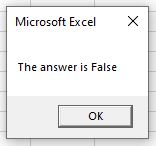As 5 is clearly not equal to 6, the result returned is False.

### Not Equal To (<>)

This operator does the opposite of the Equal To operator and check to see if the values are NOT equal.

``````Sub TestNotEqual ()
Dim Result As Boolean
Dim A As Integer
Dim B As Integer
A = 5
B = 6
Result = A<>B
Msgbox "The answer is " & Result
End Sub``````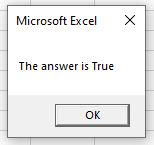So in this case the answer will be True as 5 is NOT equal to 6.

### Greater Than (>)

The Greater Than operator will check if the first value is greater than the second value.

``````Sub TestGreaterThan ()
Dim Result As Boolean
Dim A As Integer
Dim B As Integer
A = 6
B = 6
Result = A>B
Msgbox "The answer is " & Result
End Sub``````As 6 is not greater than 6, the result would be False.

### Greater than or Equal to (>=)

The Greater Than or Equal To operator will check if the first value is greater than or equal to the second value.

``````Sub TestGreaterThanEqualTo ()
Dim Result As Boolean
Dim A As Integer
Dim B As Integer
A = 6
B = 6
Result = A>=B
Msgbox "The answer is " & Result
End Sub``````In this instance, as 6 is equal to 6, the example would return True.

### Less Than (<)

The Less Than operator does the opposite of the Greater Than Operator and tests to see if the first value is less than the second value.

``````Sub TestLessThan ()
Dim Result As Boolean
Dim A As Integer
Dim B As Integer
A = 6
B = 6
Result = A<B
Msgbox "The answer is " & Result
End Sub``````So in this case, keeping the same values of 6 and 6, the result is once again False as 6 is not less than 6.

### Less Than or Equal To (<=)

The Less Than or Equal To operator will check if the first value is less than or equal to the second value.

``````Sub TestLessThanEqualTo ()
Dim Result As Boolean
Dim A As Integer
Dim B As Integer
A = 6
B = 6
Result = A<=B
Msgbox "The answer is " & Result
End Sub``````

The result of which is of course True as 6 is equal to 6!

## Logical Operators

Logical Operators also return a true or false result.  They allow us to combine 2 or more comparison operators.

 Logical Operator Explanation AND Tests to see if BOTH conditions are true OR Tests to see if ONE of the conditions is True NOT Tests to see if a condition if true, but if it is True, will return False XOR A combination of NOT and OR logical operators –  will return true if one of the conditions is true.

### AND

This allows us to use more than one comparison operator and check that both the conditions tested are true.

``````Sub TestAnd ()
Dim Result As Boolean
Dim A As Integer
Dim B As Integer
Dim C As Integer
A = 5
B = 6
C = 7
If A > B And C > B Then
Result = True
Else
Result = False
End If
MsgBox "The answer is " & Result
End Sub
``````

In this instance, as only one condition is true, the result will be False.

### OR

This allows us to use more than one comparison operator and check that one of the conditions tested are true.

``````Sub TestOR ()
Dim Result As Boolean
Dim A As Integer
Dim B As Integer
Dim C As Integer
A = 5
B = 6
C = 7
If A > B And C > B Then
Result = True
Else
Result = False
End If
MsgBox "The answer is " & Result
End Sub``````

In this instance, as one of the conditions is true (7 is greater than 6), the result will be True.

### NOT

This will test to see if a condition is True, and if it is True, it will return False, of if the condition is False, it will return True.

``````Sub TestNOT ()
Dim Result As Boolean
Dim A As Integer
Dim B As Integer
Dim C As Integer
A = 8
B = 6
C = 7
If A > B And Not C > B Then
Result = True
Else
Result = False
End If
MsgBox "The answer is " & Result
End Sub``````

In this instance, as 8 is greater than 6 and 7 is also greater than 6, the AND operator would have returned True.  However adding in the NOT operator means that the result returned is FALSE.

### XOR

This operator is the combination of NOT and OR. It is also called logical exclusion. This will return true only if one of the conditions equals true otherwise it returns false.

``````Sub TestXOR ()
Dim Result As Boolean
Dim A As Integer
Dim B As Integer
A = 7
B = 8

If A > 0 XOR B > 0 Then
Result = True
Else
Result = False
End If
MsgBox "The answer is " & Result
End Sub``````

## Concatenation Operators

Concatenation allows us to add 2 of more values together, or to combine strings of text.

 Logical Operator Explanation + Is the same as the Addition operator and will add two values or two strings of text & Will join together 2 strings of text

``````Sub ConcatenatedStrings ()
Dim A As String
Dim B As String
A = "Hello"
B = "World"
MsgBox A + " " + B
End Sub``````

The example above will give us a message box combining string A and string B, and adding a space between them – this is done using the addition operator (we are adding the strings together).

``````Sub ConcatenatedStrings ()
Dim A As String
Dim B As String
A = "Hello"
B = "World"
MsgBox A & " " & B
End Sub``````

This example above will do exactly the same thing!  However, we are using the ampersand (&) operator.

If we amend the code that uses the  above to use numbers, it will add the 2 numbers together as in the Arithmetic Operators above

``````Sub AddNumbers ()
Dim A As Integer
Dim B As Integer
A = 5
B = 6
MsgBox A + B
End Sub``````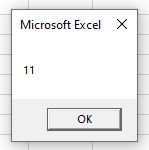However, if we use the ampersand (&) operator to do this, we will get a very different result!

``````Sub AddNumbers ()
Dim A As Integer
Dim B As Integer
A = 5
B = 6
MsgBox A & B
End Sub``````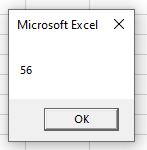This is due to the fact that the ampersand (&) operator reads the 5 and the 6 as strings (even through we have declared them to be integers)  and literally joins them together!